Author: Tal Galili ( Tal.Galili@gmail.com )

# Introduction

As mentioned in CRAN Task View: Probability Distributions

Empirical distribution : Base R provides functions for univariate analysis: (1) the empirical density (see density()), (2) the empirical cumulative distribution function (see ecdf()), (3) the empirical quantile (see quantile()) and (4) random sampling (see sample()).

This package aims to easily wrap these into a single function edfun (short for Empirical Distribution FUNctions). Also, since quantile is generally a slow function to perform, the default for creating a quantile function (inverse-CDF) is by approximating the function of predicting the data values (x) from their quantiles (CDF). This is done using the approxfun function. It takes a bit longer to create qfun, but it is MUCH faster to run than quantile (and is thus much better for simulations). Special care is taken for dealing with the support of the distribution (if it is known upfront).

The added speed allows to use these functions to run simulation studies for unusual distributions.

# Installation

To install the stable version on CRAN:

# install.packages('edfun') # not on CRAN yet

To install the GitHub version:

# You'll need devtools
if (!require(devtools)) install.packages("devtools");

devtools::install_github('talgalili/edfun')

And then you may load the package using:

library("edfun")

# Usage

## Basic usage - the normal distribution

library(edfun)
set.seed(123)
x <- rnorm(1000)
x_dist <- edfun(x)

f <- x_dist$dfun curve(f, -2,2)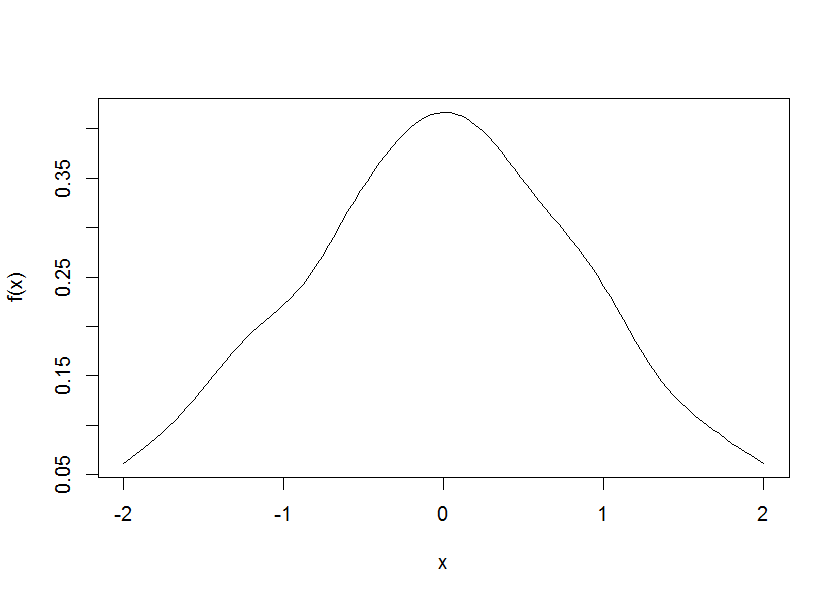f <- x_dist$pfun
curve(f, -2,2)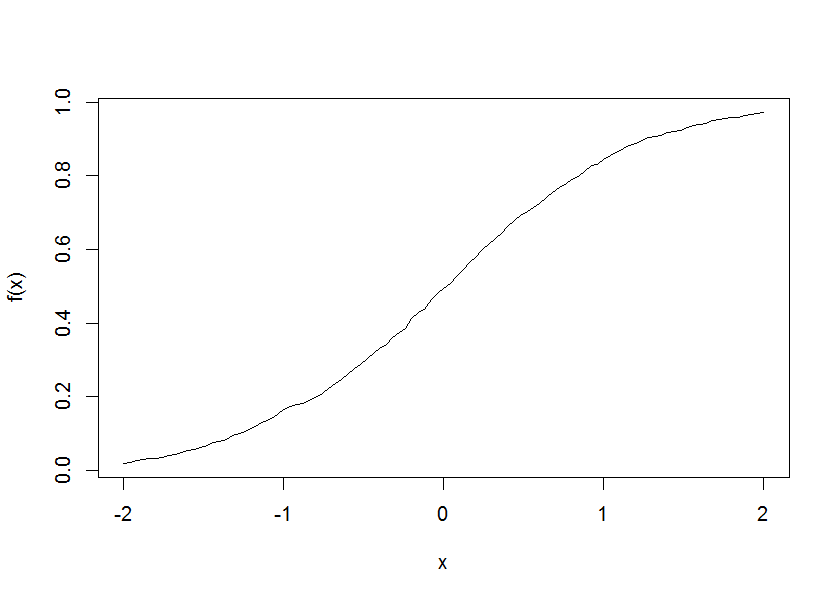f <- x_dist$qfun curve(f, 0,1)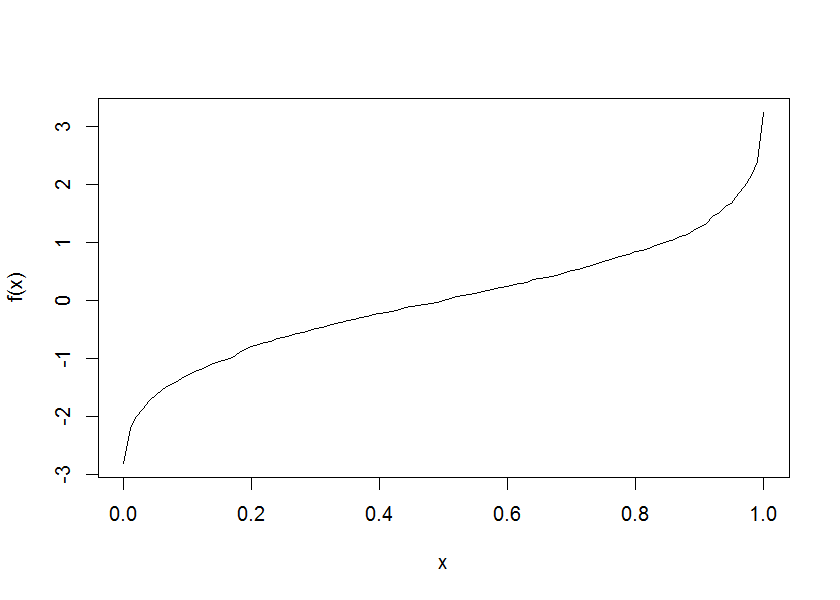new_x <- x_dist$rfun(1000)
hist(new_x)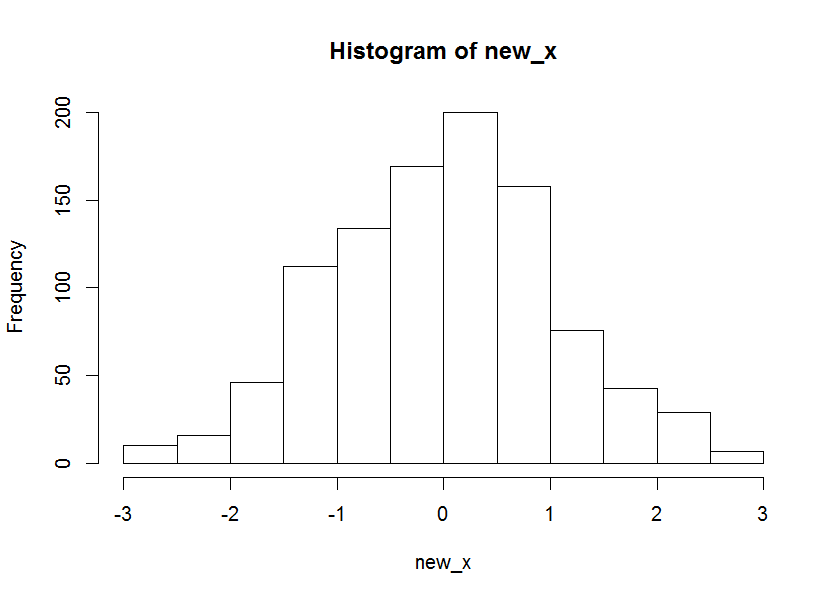The same can be done if we had the density of the distribution. In this case, x should be a sequence of values for which we wish to pre-compute the quantiles (inv-CDF).

x <- seq(-4,4, length.out = 1e3)
x_dist <- edfun(x, dfun = dnorm)

f <- x_dist$dfun curve(f, -2,2)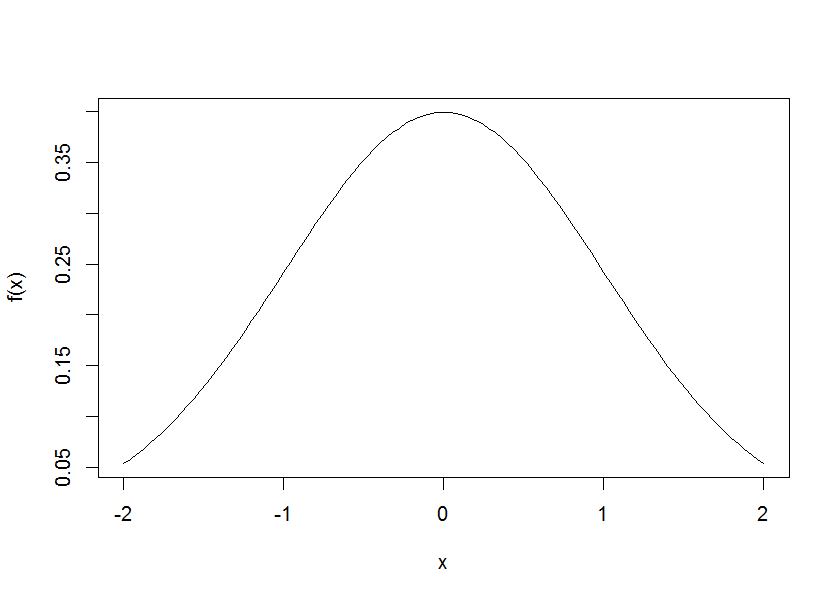f <- x_dist$pfun
curve(f, -2,2)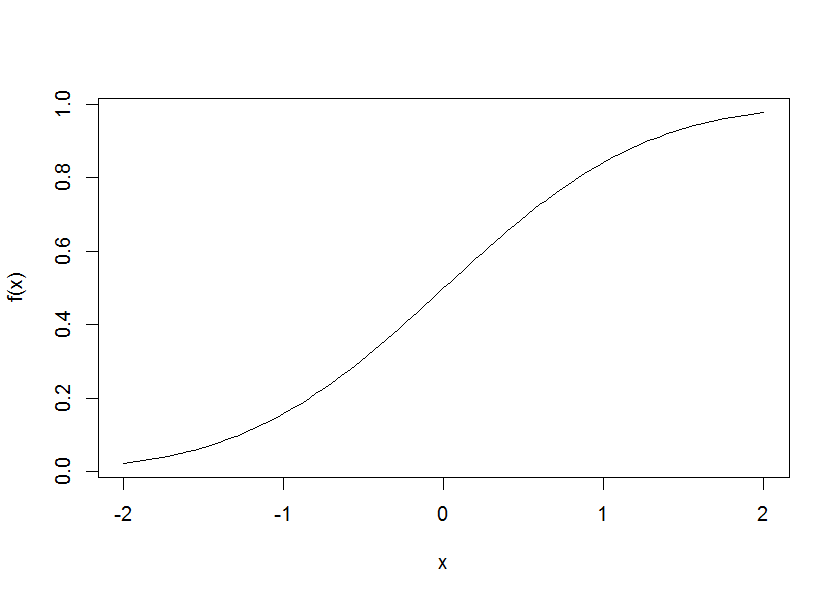f <- x_dist$qfun curve(f, 0,1)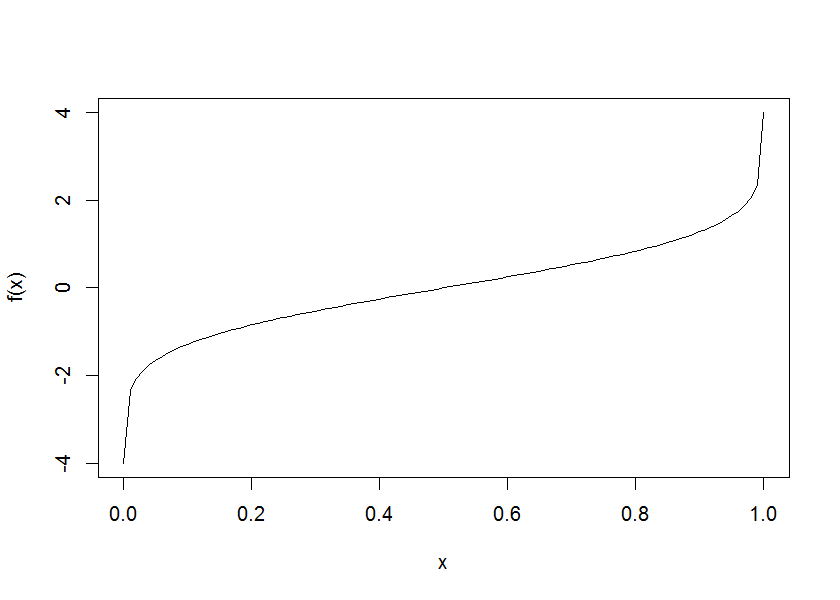new_x <- x_dist$rfun(1000)
hist(new_x)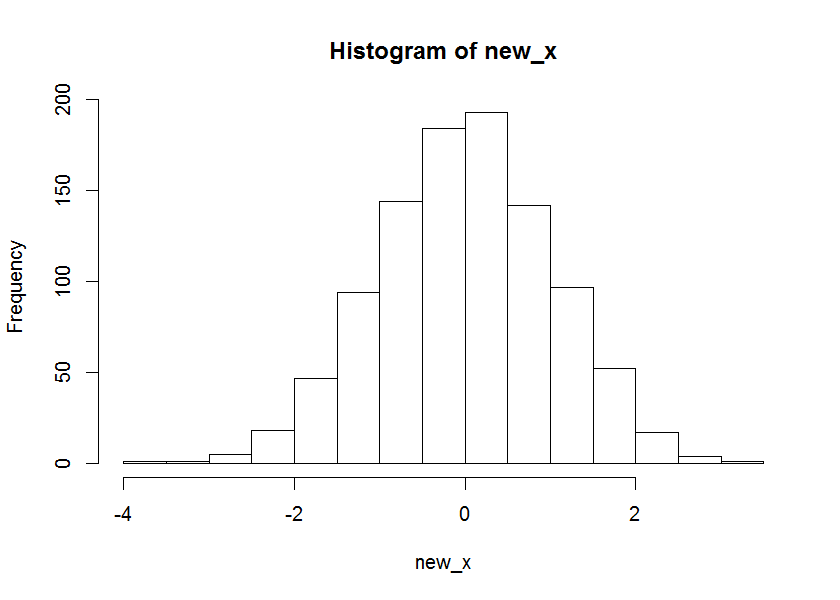If dfun is NULL then it is just returned as NULL. This is useful if using the functions ONLY for creating the CDF and inv-CDF and wanting to save computation time.

set.seed(123)
x <- rnorm(1000)
x_dist <- edfun(x, dfun = NULL)

x_dist$dfun #> NULL # but this still works just fine: f <- x_dist$pfun
curve(f, -2,2)f <- x_dist$qfun curve(f, 0,1)If it is known, we can specifically define the support: x <- seq(-4,4, length.out = 1e3) x_dist_no_support <- edfun(x, dfun = dnorm) x_dist_known_support <- edfun(x, dfun = dnorm, support = c(-Inf, Inf)) x_dist_no_support$qfun(0)
#>  -4
x_dist_known_support$qfun(0) #>  -Inf Timing is basically the same when using the functions relying on density (i.e.: dfun) or on a sample only. But the accuracy of using the density is much better.  set.seed(2016-08-18) x_simu <- rnorm(1e5) x_funs_simu <- edfun(x_simu, support = c(-Inf, Inf)) x <- seq(-4,4, length.out = 1e5) x_funs <- edfun(x, dfun = dnorm, support = c(-Inf, Inf)) x_funs$qfun(0) # -Inf

# precision - qfun
q_to_test <- seq(0.001,.999, length.out = 100)
edfun_out <- x_funs$qfun(q_to_test) # -4 edfun_simu_out <- x_funs_simu$qfun(q_to_test) # -4
real_out <- qnorm(q_to_test)

mean(abs(edfun_out-real_out))
mean(abs(edfun_simu_out-real_out)) # quite terrible compared to when knowing dfun

library(microbenchmark)
microbenchmark(dfun_known = x_funs$qfun(q_to_test), dfun_NOT_known = x_funs_simu$qfun(q_to_test))
# same time for the q function!

# precision - pfun
q_to_test <- seq(-3,3, length.out = 100)
edfun_out <- x_funs$pfun(q_to_test) # -4 edfun_simu_out <- x_funs_simu$pfun(q_to_test) # -4
real_out <- pnorm(q_to_test)

mean(abs(edfun_out-real_out))
mean(abs(edfun_simu_out-real_out)) # quite terrible compared to when knowing dfun

library(microbenchmark)
microbenchmark(dfun_known = x_funs$pfun(q_to_test), dfun_NOT_known = x_funs_simu$pfun(q_to_test))
# same time for the p function!

# timing for the rfun
library(microbenchmark)
microbenchmark(dfun_known = x_funs$rfun(100), dfun_NOT_known = x_funs_simu$rfun(100))
# this rfun is slower...  

## The double exponential distribution

library(edfun)
# credit: library(smoothmest)
ddoublex <- function (x, mu = 0, lambda = 1) {
exp(-abs(x - mu)/lambda)/(2 * lambda)
}

curve(ddoublex, -4,4) # the peak in 0 should go to Inf, but it doesn't because of the limits of curve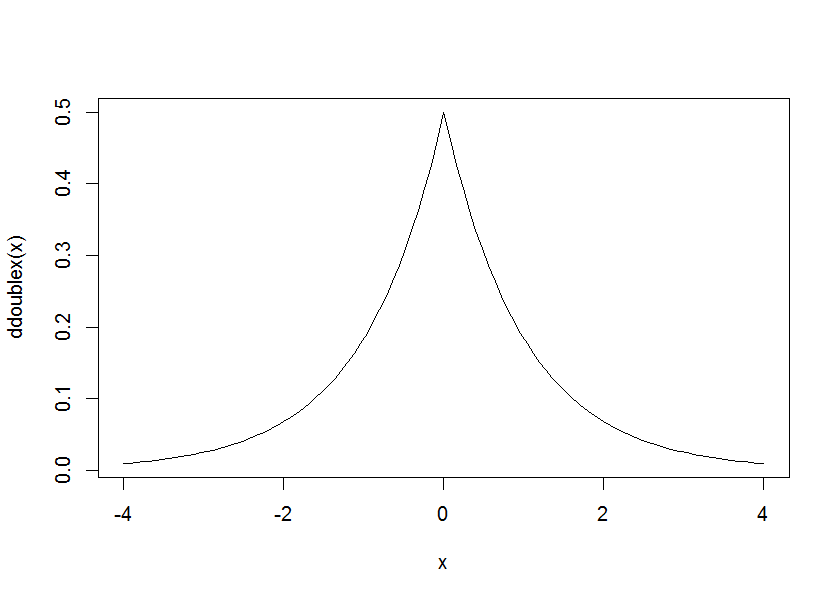x <- seq(-4,4, length.out = 1e3)
x_dist <- edfun(x, dfun = ddoublex)

f <- x_dist$dfun curve(f, -4,4)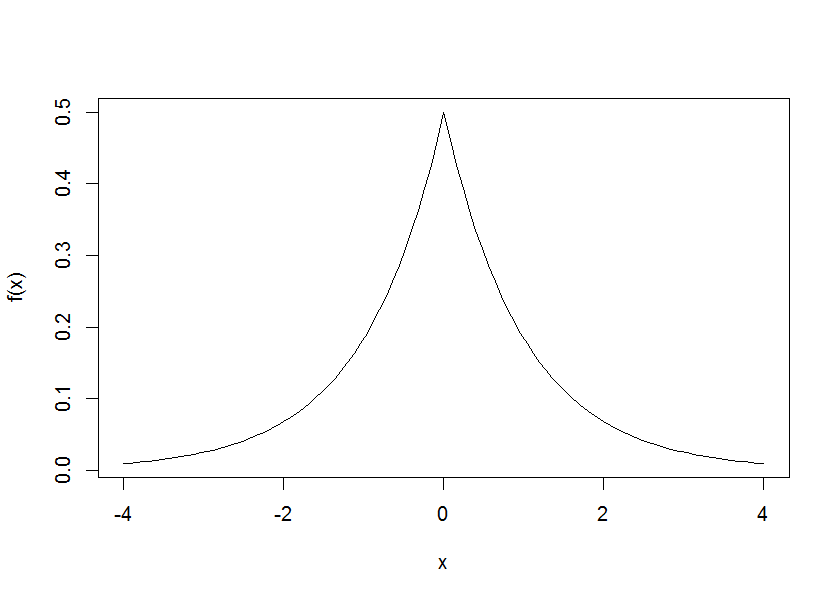f <- x_dist$pfun
curve(f, -4,4)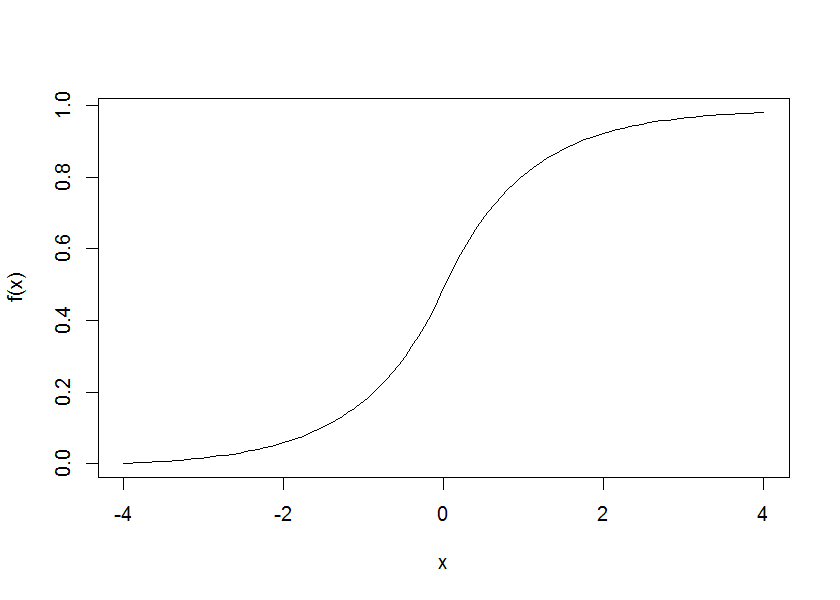f <- x_dist$qfun curve(f, 0,1)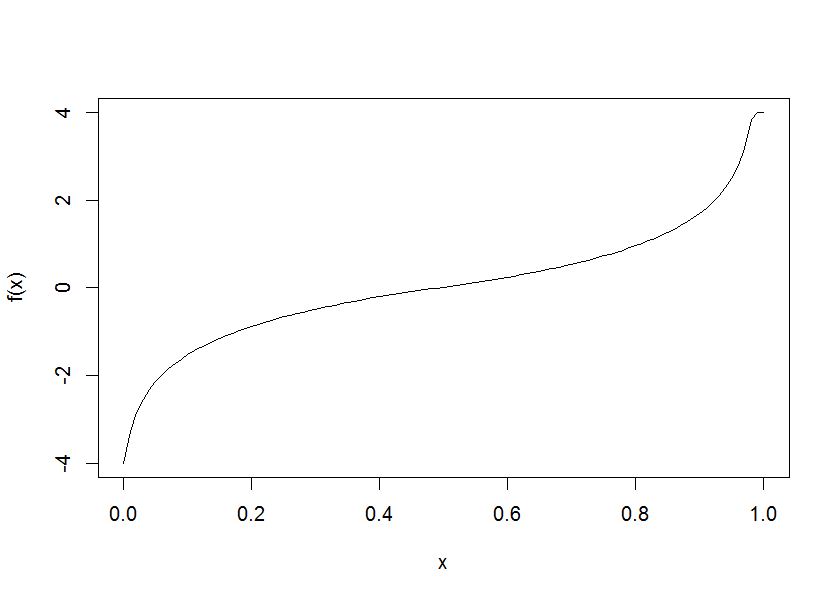new_x <- x_dist$rfun(1000)
hist(new_x)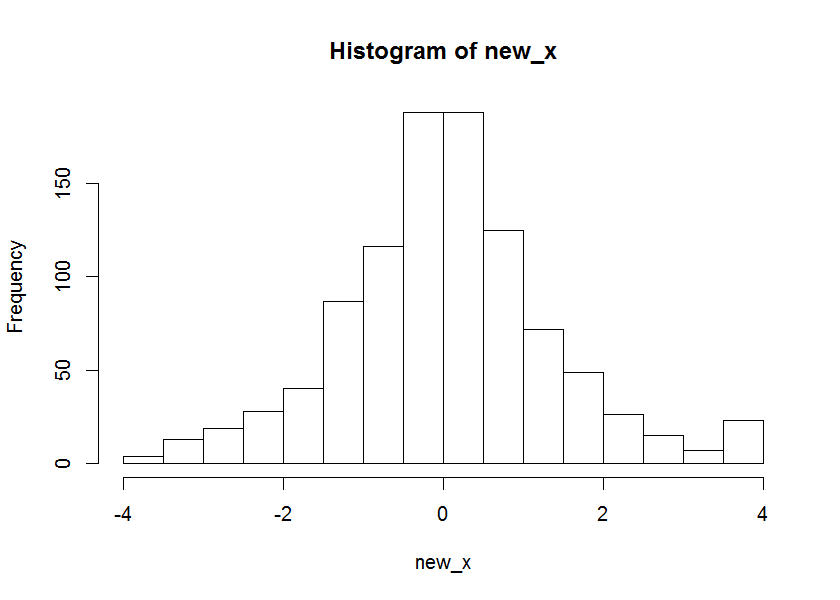## A mixture of two normal distributions

library(edfun)
# http://stackoverflow.com/questions/20452650/writing-a-bimodal-normal-distribution-function-in-r?rq=1
# random sample:
# mixtools::rnormmix # random sample of a mixture of distributions
# http://stats.stackexchange.com/questions/70855/generating-random-variables-from-a-mixture-of-normal-distributions
# nor1mix::rnorMix
# https://en.wikipedia.org/wiki/Mixture_distribution
# density:
dmixnorm <- function(x, p1 = 0.5, m1=0, m2=0, s1=1, s2=1) {
p1 * dnorm(x, m1, s1) + (1-p1) * dnorm(x, m2, s2)
}
# a convex mixture of densities is a density:
#  https://en.wikipedia.org/wiki/Mixture_distribution#Properties

# yay - it works.
dmixnorm_1 <- function(x) dmixnorm(x, .75, 0,5,  1,1)
curve(dmixnorm_1, -4,9)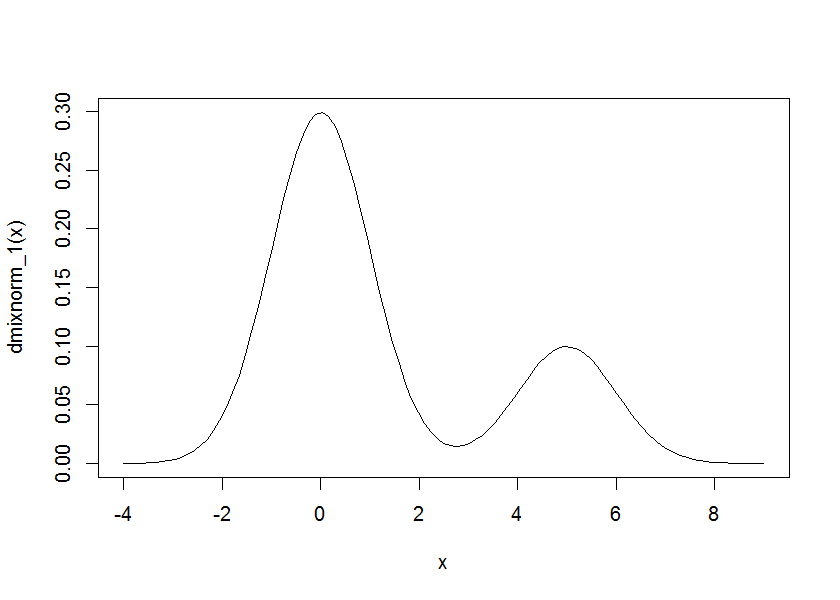x <- seq(-4,9, length.out = 1e3)
x_dist <- edfun(x, dfun = dmixnorm_1)

f <- x_dist$dfun curve(f, -4,9)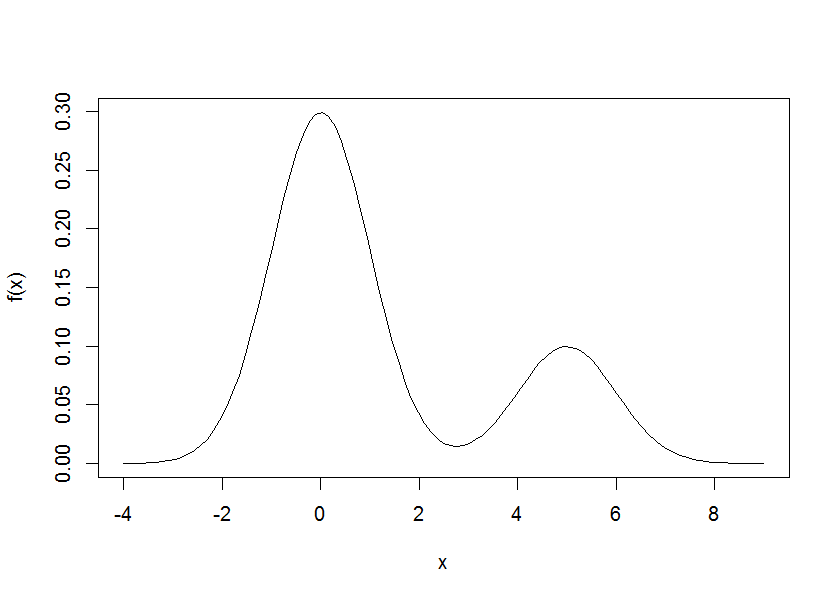f <- x_dist$pfun
curve(f, -4,9)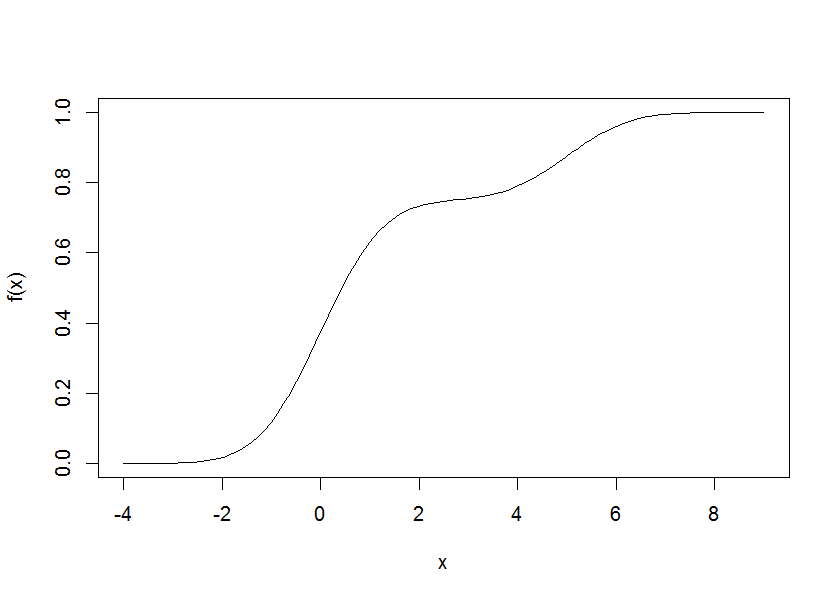f <- x_dist$qfun curve(f, 0,1)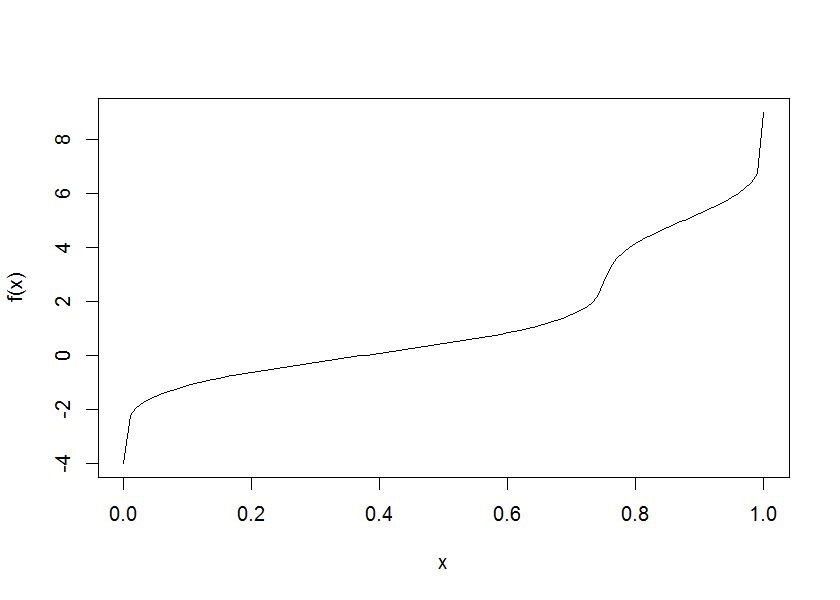new_x <- x_dist$rfun(1000)
hist(new_x)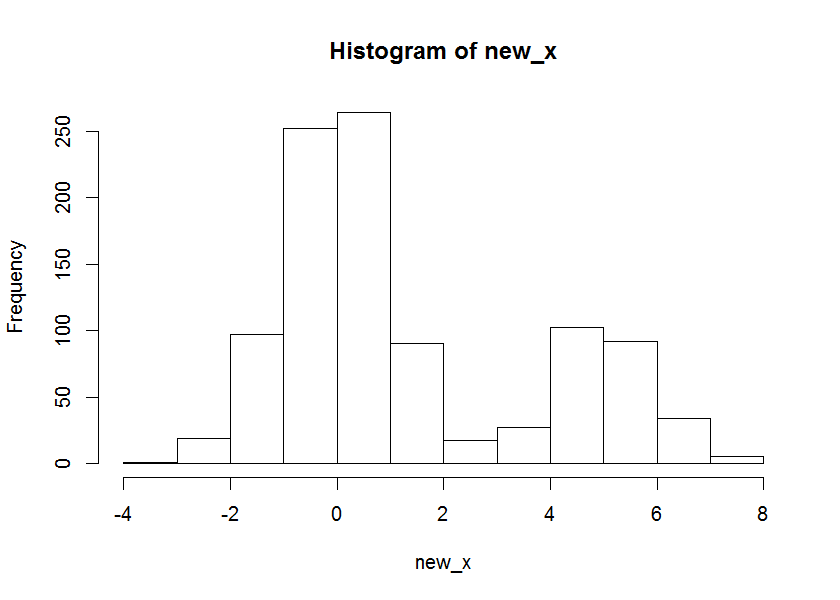# Contact

You are welcome to:

# Latest news

You can see the most recent changes to the package in the NEWS.md file

# Session info

sessionInfo()
#> R version 3.3.0 (2016-05-03)
#> Platform: x86_64-w64-mingw32/x64 (64-bit)
#> Running under: Windows 7 x64 (build 7601) Service Pack 1
#>
#> locale:
#>  LC_COLLATE=C                   LC_CTYPE=Hebrew_Israel.1255
#>  LC_MONETARY=Hebrew_Israel.1255 LC_NUMERIC=C
#>  LC_TIME=Hebrew_Israel.1255
#>
#> attached base packages:
#>  stats     graphics  grDevices utils     datasets  methods   base
#>
#> other attached packages:
#>  knitr_1.13  edfun_0.2.0
#>
#> loaded via a namespace (and not attached):
#>   magrittr_1.5    formatR_1.4     htmltools_0.3.5 tools_3.3.0
#>   yaml_2.1.13     Rcpp_0.12.5     rmarkdown_0.9.6 stringi_1.1.1
#>   digest_0.6.9    stringr_1.0.0   evaluate_0.9